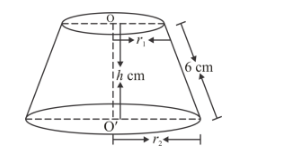# If the slant height of the frustum of a cone is 6 cm`
Question:

If the slant height of the frustum of a cone is 6 cm and the perimeters of its circular bases are 24 cm and 12 cm respectively. What is the curved surface area of the frustum?

Solution:The parameter of upper base

$=2 \pi r_{1}$

$2 \pi r_{1}=12$

$r_{1}=\frac{6}{\pi} \mathrm{cm}$

The parameter of lower base

$=2 \pi r_{2}$

$2 \pi r_{2}=24$

$r_{2}=\frac{12}{\pi} \mathrm{cm}$

The surface area of frustum

$=\pi\left(\frac{6}{\pi}+\frac{12}{\pi}\right) \times 6$

$=\pi \times \frac{18}{\pi} \times 6$

$=108 \mathrm{~cm}^{2}$